# Exam-Style Questions on Calculus

## Problems on Calculus adapted from questions set in previous Mathematics exams.

### 1.

IB Studies

A function is given as $$f(x)=3x^2-6x+4+\frac3x,-2\le x \le 4, x\ne 0$$.

(a) Find the derivative of the function.

(b) Find the coordinates of the local minimum point of $$f(x)$$ in the given domain using your calculator.

### 2.

IB Studies

Consider the graph of the function $$f(x)=7-3x^2-x^3$$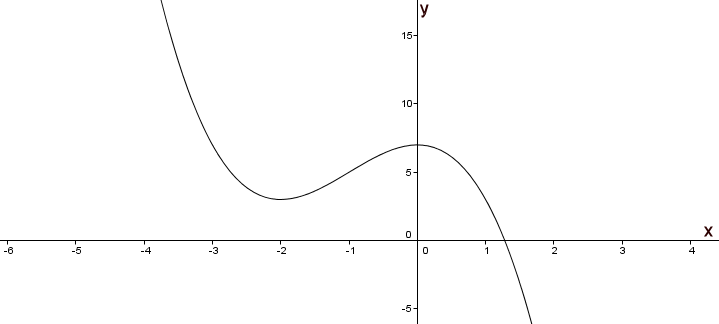(a) Label the local maximum as A on the graph.

(b) Label the local minimum as B on the graph.

(c) Write down the interval where $$f(x)>5$$.

(d) Draw the tangent to the curve at $$x=-3$$ on the graph.

(e) Write down the equation of the tangent at $$x=-3$$.

### 3.

IB Standard

The graph of $$f(x)=8-x^2$$ crosses the x-axis at the points A and B.

(a) Find the x-coordinate of A and of B.

(b) The region enclosed by the graph of $$f$$ and the x-axis is revolved 360o about the x-axis. Find the volume of the solid formed.

### 4.

IB Standard

The acceleration, $$a$$ ms-2 , of an object at time $$t$$ seconds is given by

$$a=\frac1t+4sin3t, (t\ge1)$$

The object is at rest when $$t=1$$.

Find the velocity of the object when $$t=7$$.

### 5.

IB Standard

Let $$f(x)=\frac{2x}{x^2+3}$$

(a) Use the quotient rule to show that $$f'(x)=\frac{6-2x^2}{(x^2+3)^2}$$.

(b) Find $$\int \frac{2x}{x^2+3}dx$$.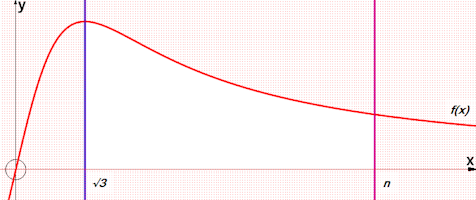(c) The area enclosed by the graph of $$f(x)$$, the x-axis and the lines $$x=\sqrt3$$ and $$x=n$$ has an area of $$\ln14$$. Find the value of $$n$$.

### 6.

IB Analysis and Approaches

Find:

$$\int^{16}_9 \frac{6-2\sqrt{x}}{\sqrt{x}} dx$$

by first writing the algebraic fraction in the form $$ax^b+c$$.

### 7.

IB Analysis and Approaches

(a) Find $$\int (4x+5) dx$$.

(b) Given $$f'(x) = 4x+5$$ find $$f(x)$$ if $$f(3.4) = 10.12$$.

### 8.

IB Analysis and Approaches

Given that $$\frac{dy}{dx} = \sin(x + \frac{\pi}{3})$$ and $$y = 5$$ when $$x = \frac{8\pi}{3}$$, find $$y$$ in terms of $$x$$.

### 9.

A-Level

(a) Find $$\frac{dy}{dx}$$ when:

$$y= (7-5x^2)^{ \frac12 }$$

(b) Find the following integral:

$$\int (1 - cos3x) dx$$

### 10.

IB Analysis and Approaches

A particle moves in a straight line. During the first nine seconds the velocity, $$v$$ ms-1 of the particle at time $$t$$ seconds is given by:

$$v(t) = t \cos(t+5)$$

The following diagram shows the graph of v: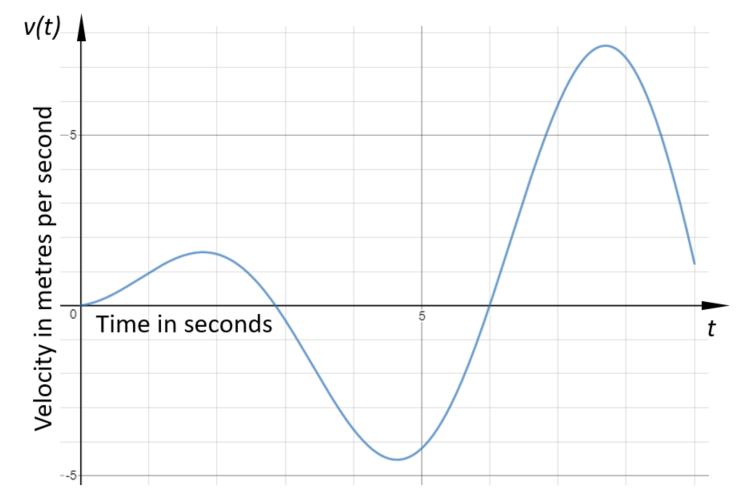(a) Find the maximum value of $$v$$.

(b) Find the acceleration of the particle when t = 6.

(c) Find the total distance travelled by the particle.

### 11.

IB Analysis and Approaches

The diagram below shows part of the graph of $$y = \dfrac{2x}{9-x^2}$$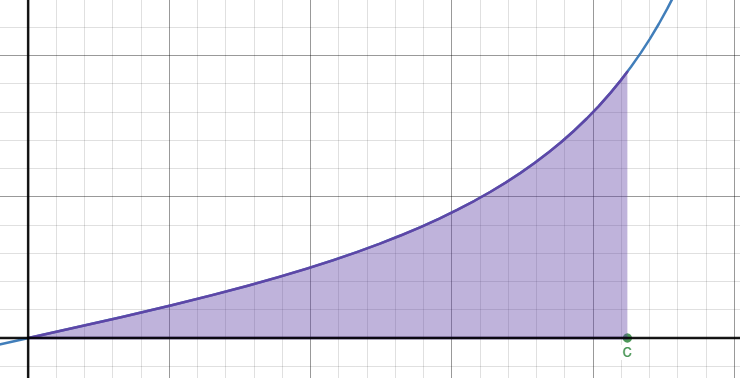The shaded region is bounded by the curve, the x-axis and the line $$x = c$$

The area of this region is $$\ln{2}$$

Find the value of $$c$$.

### 12.

IB Studies

Consider the function $$f(x)=6 - ax+\frac 3{x^2},x\neq 0$$

(a) Write down the equation of the vertical asymptote of the graph of $$y=f(x)$$.

(b) Write down $$f'(x)$$

The line T is the tangent to the graph of $$y=f(x)$$ at the point where $$x=1$$ and it has a gradient of -8.

(c) Show that $$a=2$$.

(d) Find the equation of T.

(e) Using your calculator find the coordinates of the point where the graph of $$y=f(x)$$ intersects the x-axis.

(f) The line T also intersects $$f(x)$$ when $$-2\le x\le 0$$. Find the coordinates of this intersection.

### 13.

IB Analysis and Approaches

Consider the function $$f$$ defined by $$f(x)= \ln{(x^2 - 9)}$$ for $$x > 3$$.

The following diagram shows part of the graph of $$f$$ which crosses the x-axis at point $$A$$ with coordinates $$(a,0)$$.

The line $$L$$ is the tangent to the graph of $$f$$ at the point $$B$$ with coordinates $$(b,c)$$. The gradient of $$L$$ is $$\frac14$$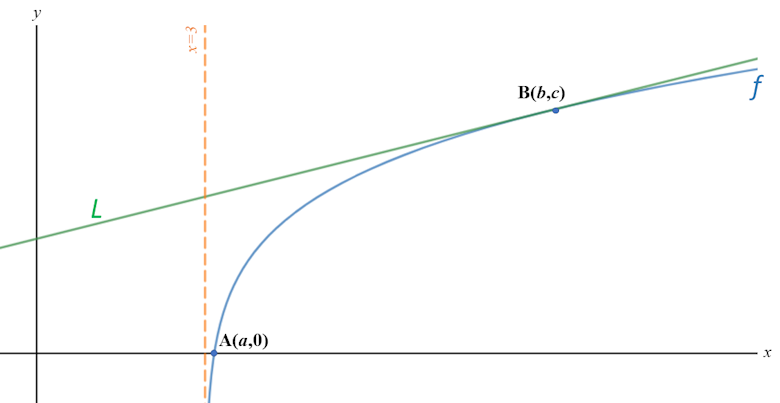(a) Find the exact value of $$a$$.

(b) Find the value of $$b$$.

### 14.

IB Standard

If $$f(x)=x\sin{x}$$, for $$-3\le x\le3$$

(a) Find $$f'(x)$$

(b) Sketch the graph of $$y=f'(x)$$, for $$-3\le x\le3$$

### 15.

IB Analysis and Approaches

The function $$f$$ is defined by $$f(x) = 8 - 5 \sin{x}$$, for $$x \ge 0$$.

The diagram shows part of the graph of $$y = f (x)$$.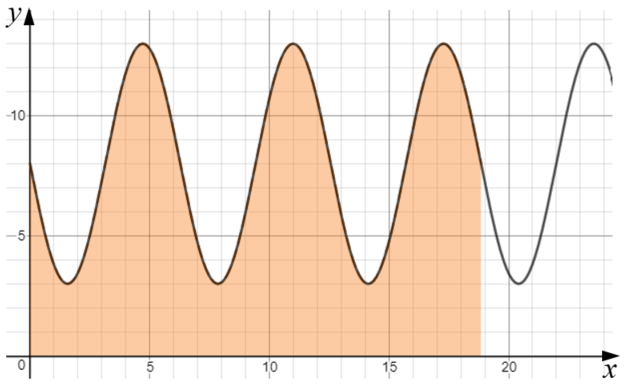The shaded region is enclosed by the graph of $$y=f(x)$$ and the x-axis for the first three periods of the function.

(a) Find the exact value of the x-coordinate of the right side of the shaded region.

(b) Show that the area of the shaded region is $$48 \pi$$.

A hemisphere has a total surface area in square centimetres equal to the shaded area in the previous diagram.

(c) Find the radius of the hemisphere.

### 16.

IB Analysis and Approaches

The function $$f$$ is defined for all $$x \in \mathbb{R}$$. The line with equation $$y=5x+3$$ is the tangent to the graph of $$f$$ at $$x = 2$$

(a) Write down the value of $$f'(2)$$.

(b) Find $$f(2)$$.

The function $$g$$ is defined for all $$x \in \mathbb{R}$$ where $$g(x) = x^3 - 3x$$ and $$h(x) = f(g(x))$$.

(c) Find $$h(2)$$.

(d) Hence find the equation of the tangent to the graph of $$h$$ at $$x = 2$$.

### 17.

IB Analysis and Approaches

Consider the cubic function $$f(x)=\frac{1}{6}x^3-2x^2+6x-2$$

(a) Find $$f'(x)$$

The graph of $$f$$ has horizontal tangents at the points where $$x = a$$ and $$x = b$$ where $$a < b$$.

(b) Find the value of $$a$$ and the value of $$b$$

(c) Sketch the graph of $$y = f'(x)$$.

(d) Hence explain why the graph of $$f$$ has a local maximum point at $$x = a$$.

(e) Find $$f''(b)$$.

(f) Hence, use your answer to part (e) to show that the graph of $$f$$ has a local minimum point at $$x = b$$.

(g) Find the coordinates of the point where the normal to the graph of $$f$$ at $$x = a$$ and the tangent to the graph of $$f$$ at $$x = b$$ intersect.

### 18.

IB Applications and Interpretation

The following diagram shows part of the graph of:

$$f (x) = \frac{(9 + 4x) (6 - x)}{10}, x \in \mathbf R$$

The shaded region A is bounded by the x-axis, y-axis and the graph of $$f$$.(a) Write down an integral for the area of region A.

(b) Find the area of region A.

The three points A (0 , 0) , B (6 , 9) and C (p , 0) define the vertices of a triangle.

(c) Find the value of p, the x-coordinate of C , such that the area of the triangle is equal to the area of region A.

### 19.

IB Studies

Consider the function $$f(x)=x^3-9x+2$$.

(a) Sketch the graph of $$y=f(x)$$ for $$-4\le x\le 4$$ and $$-14\le y\le 14$$ showing clearly the axes intercepts and local maximum and minimum points. Use a scale of 2 cm to represent 1 unit on the x-axis, and a scale of 1 cm to represent 2 units on the y-axis.

(b) Find the value of $$f(-1)$$.

(c) Write down the coordinates of the y-intercept of the graph of $$f(x)$$.

(d) Find $$f'(x)$$.

(e) Find $$f'(-1)$$

(f) Explain what $$f'(-1)$$ represents.

(g) Find the equation of the tangent to the graph of $$f(x)$$ at the point where x is –1.

R and S are points on the curve such that the tangents to the curve at these points are horizontal. The x-coordinate of R is $$a$$ , and the x-coordinate of S is $$b$$ , $$b \gt a$$.

(h) Write down the value of $$a$$ ;

(i) Write down the value of $$b$$.

(j) Describe the behaviour of $$f(x)$$ for $$a \lt x \lt b$$.

### 20.

IB Standard

Consider the graph of the function $$f(x)=x^2+2$$.

(a) Find the area between the graph of $$f$$ and the x-axis for $$2\le x \le 3$$.

(b) If the area described above is rotated 360o around the x-axis find the volume of the solid formed.

### 21.

IB Standard

Let $$f(x)=\frac{g(x)}{h(x)}$$, where $$g(3)=36$$, $$h(3)=12$$, $$g'(3)=10$$ and $$h'(3)=4$$. Find the equation of the normal to the graph of $$f$$ at $$x=3$$.

### 22.

A-Level

(a) Express the algebraic fraction

$$\frac{6x^2 - 47x + 49}{(5-x)(1-2x)}$$

in the form

$$A + \frac{B}{5-x} + \frac{C}{1-2x}$$

where $$A$$, $$B$$ and $$C$$ are integers.

(b) Hence show that the following integral equates to 3.03 correct to three significant figures.

$$\int^{0.25}_0 \frac{6x^2 - 47x + 49}{(5-x)(1-2x)} dx$$

### 23.

IB Analysis and Approaches

The displacement, in millimetres, of a particle from an origin, O, at time t seconds, is given by $$s(t) = t^3 cos t + 5t sin t$$ where $$0 \le t \le 5$$ .

(a) Find the maximum distance of the particle from O.

(b) Find the acceleration of the particle at the instant it first changes direction.

### 24.

IB Standard

The function $$f$$ is defined as follows:

$$f(x)=\frac{122}{1+60e^{-0.3x}}$$

(a) Calculate $$f(0)$$.

(b) Find a value of $$x$$ for which $$f(x)=85$$

(c) Find the range of $$f$$.

(d) Show that:

$$f'(x)=\frac{2196e^{-0.3x}}{(1+60e^{-0.3x})^2}$$

(e) Find the maximum rate of change of $$f$$.

### 25.

IB Analysis and Approaches

Consider the functions

$$f(x) = m-x^2 - 2xn - n^2$$ $$g(x) = \frac{e^x}{e^2} + \frac{m}{2}$$

where $$m, n \in \mathbb R$$

(a) Find $$f'(x)$$.

(b) Find $$g'(x)$$.

The graphs of $$f$$ and $$g$$ have a common, non-vertical tangent at $$x=3$$.

(c) Find expressions for $$m$$ and $$n$$ in terms of $$e$$.

### 26.

IB Standard

The following diagram shows part of the graph of $$y=f (x)$$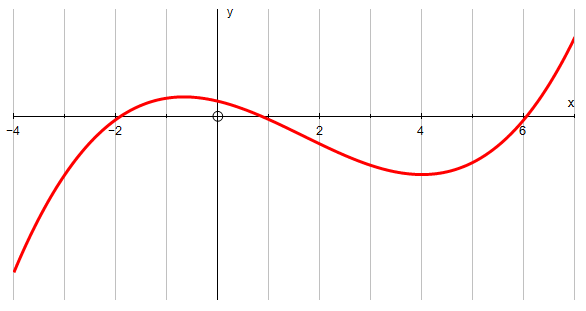The graph has a local maximum where $$x=- \frac23$$, and a local minimum where $$x=4$$.

sketch the graph of $$y=f'(x)$$ for $$-4\le x \le 7$$

Write down the following in order from least to greatest: $$f(2),f'(4)$$ and $$f''(4)$$.

### 27.

IB Standard

Make a sketch of a graph showing the velocity (in $$ms^{-1}$$) against time of a particle travelling for six seconds according to the equation:

$$v=e^{\sin t}-1$$

(a) Find the point at which the graph crosses the $$t$$ axis.

(b) How far does the particle travel during these first six seconds?

### 28.

IB Standard

Find the value of $$a$$ if $$\pi \lt a \lt 2\pi$$ and:

$$\int_\pi^a sin3x dx = -\frac13$$

### 29.

IB StandardThis graph represents the function $$f:x\to a \cos x, a\in \mathbf N$$

(a) Find the value of $$a$$.

(b) Find the area of the shaded region.

### 30.

IB Standard

Find $$f(x)$$ if $$f'(x)=6 \sin2x$$ and the graph of $$f(x)$$ passes through the point $$(\frac{\pi}{3},11)$$.

### 31.

IB Standard

A particle P moves along a straight line. The velocity $$v$$ in metres per second of P after $$t$$ seconds is given by $$v(t) = 3\sin{t} - 8t^{\cos{t}}, 0 \le t \le 7$$.

(a) Find the initial velocity of P.

(b) Find the maximum speed of P.

(c) Write down the number of times that the acceleration of P is 0 ms-2.

(d) Find the acceleration of P at a time of 5 seconds.

(e) Find the total distance travelled by P.

### 32.

IB Analysis and Approaches

Consider the functions $$f(x)=\sin(x) \text{ and } g(x) = \tan(x+\frac{\pi}{2})$$ in the region where $$\frac{\pi}{2}\le x \le \pi$$

The graphs $$y=f (x)$$ and $$y = g(x)$$ intersect at a point A whose x-coordinate is $$a$$.

(a) Show that $$\sin^2{a}=-\cos{a}$$.

(b) Hence, show that the tangents to the curves at A intersect at right angles.

Find the value of $$\cos(a)$$. Give your answer in the form $$\dfrac{a + \sqrt{b}}{c}$$.

### 33.

IB Analysis and Approaches

The curve $$y=\sin(\sqrt{x}) \text{ where } x \ge 0$$ intersects the x axis at the points $$x_0, x_1, x_2, x_3, x_4, ...$$ and $$x_0 = 0$$.

(a) Find $$x_1$$, the first x-intercept of the function to the right of the origin. Give your answer in terms of $$\pi$$.

(b) Find an expression for the nth x-intercept of the curve, in terms of $$\pi$$.

(c) By using an appropriate substitution, show that:

$$\int \sin(\sqrt{x}) \; dx = 2\sin(\sqrt{x}) - 2 \sqrt{x} \cos(\sqrt{x})$$

The area of the region bounded by the curve and the x-axis is denoted by $$R_n$$ where:

$$R_n = \int^{x_{n+1}}_{x_n} y \; dx$$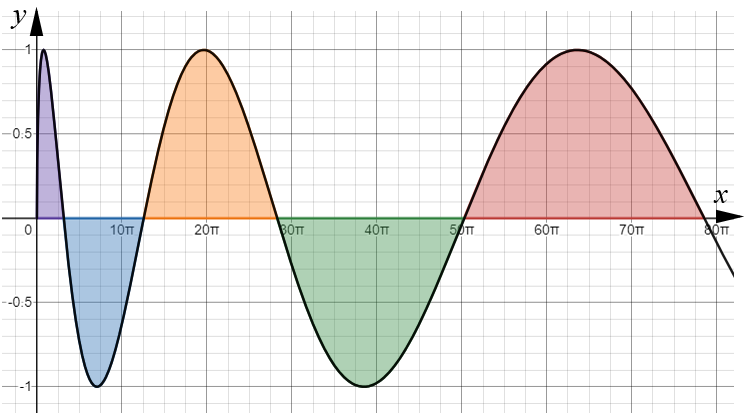(d) Calculate the area of region $$R_n$$ giving your answer in terms of $$\pi$$.

(e) Hence, show that the areas of the regions bounded by the curve and the x-axis, form an arithmetic sequence.

### 34.

A-Level

The diagram shows a sketch of the curve C with equation: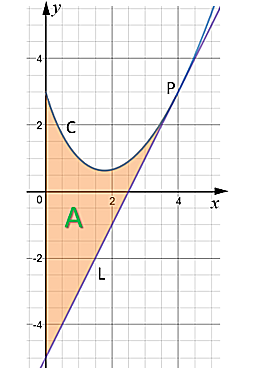$$y=2x^{\frac32}-4x+3$$

The point P with coordinates (4, 3) lies on C.

The line L is the tangent to C at the point P.

The region A, shown shaded, is bounded by the curve C, the line L and the y-axis.

Find the area of A making your method clear.

Note that solutions based entirely on graphical or numerical methods are not acceptable.)

### 35.

IB Analysis and Approaches

The function $$f$$ is such that $$f(x) = \frac{\ln2x}{x^3}$$ where $$x \gt 0$$.

(a) Find the first derivative of the function, $$f'(x)$$.

(b) The graph of $$y=f(x)$$ has a horizontal tangent at the point T. Find the coordinates of T.

(c) Show that T is a local maximum point by considering the second derivative, $$f''(x)$$.

(d) Find the values of $$x$$ for which $$f(x) \gt 0$$.

(e) Sketch the graph of $$f$$ showing clearly the value of the x-intercept and the approximate position of point T.

### 36.

IB Analysis and Approaches

Consider the function $$f$$ defined by $$f(x) = 25e^{x-5}$$ for $$x \in \mathbb{R}^+$$.

(a) Find the coordinates of the points where the graph of $$f$$ intersects the line $$y=x$$.

The line $$L$$ has a gradient of $$-1$$ and is a normal to the graph of $$f$$ at the point $$R$$.

(b) Find the exact coordinates of $$R$$.

(c) Show that the equation of the line $$L$$ is $$y=-x+6- \ln{25}$$.

(d) Find the area of the region enclosed by the graph of $$f$$ and its inverse.

### 37.

IB Standard

The following diagram shows the graph of $$f(x) = \cos(e^x) \; \text{for} \; 0 \le x \le 0.5$$.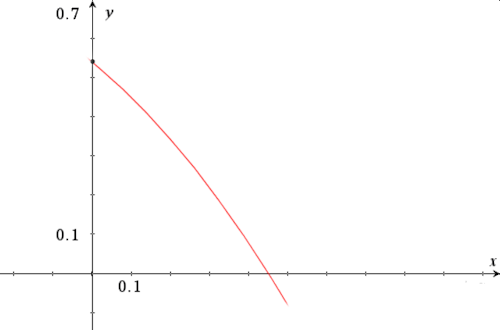(a) Find the x-intercept of the graph of $$f(x)$$.

The region enclosed by the graph of $$f(x)$$, the y-axis and the x-axis is rotated 360° about the x-axis.

(b) Find the volume of the solid formed.

### 38.

A-Level

The following equation defines a curve which passes through $$A( 2 \pi ,3 \pi)$$

$$\sin 2y + y \cos^22x = x + c$$

(a) Find the exact value of the constant $$c$$.

(b) Find an expression for $$\frac{dy}{dx}$$

(c) Find the equation of the normal to the curve at A, giving your answer in the form $$y = mx + b$$

### 39.

IB Analysis and Approaches

Find $$f(x)$$ if:

$$f'(x) = \frac{12x}{\sqrt{3x^2+4}}$$

given that $$f(0) = 10$$

### 40.

IB Analysis and Approaches

Let $$f(x) = \frac{ln3x}{kx}$$ where $$x \gt 0$$ and $$k \in \mathbf Q^+$$.

(a) Find an expression for the first derivative $$f'(x)$$.

The graph of $$f$$ has exactly one maximum point at P.

(b) Find the x-coordinate of P.

The graph of $$f$$ has exactly one point of inflection at Q.

(c) Find the x-coordinate of Q.

(d) The region enclosed by the graph of $$f$$, the x-axis, and the vertical lines through P and Q has an area of one square unit, find the value of $$k$$.

### 41.

IB Studies

Consider the function $$f(x)=\frac{20}{x^2}+kx$$ where $$k$$ is a constant and $$x\neq0$$.

(a) Write down $$f'(x)$$

The graph of $$y = f(x)$$ has a local minimum point at $$x=2$$.

(b) Show that $$k=5$$.

(c) Find $$f(1)$$.

(d) Find $$f'(1)$$.

(e) Find the equation of the normal to the graph of $$y=f(x)$$ at the point where $$x=1$$
Give your answer in the form $$ay+bx+c=0$$ where $$a, b, c \in \mathbb{Z}$$

(f) Sketch the graph of $$y=f(x)$$ , for $$-5\le x\le 10$$ and $$-10\le y\le 50$$.

(g) Write down the coordinates of the point where the graph of $$y=f(x)$$ intersects the x-axis.

(h) State the values of $$x$$ for which $$f(x)$$ is decreasing.

If you would like space on the right of the question to write out the solution try this Thinning Feature. It will collapse the text into the left half of your screen but large diagrams will remain unchanged.

The exam-style questions appearing on this site are based on those set in previous examinations (or sample assessment papers for future examinations) by the major examination boards. The wording, diagrams and figures used in these questions have been changed from the originals so that students can have fresh, relevant problem solving practice even if they have previously worked through the related exam paper.

The solutions to the questions on this website are only available to those who have a Transum Subscription.

Exam-Style Questions Main Page

Search for exam-style questions containing a particular word or phrase:

To search the entire Transum website use the search box in the grey area below.